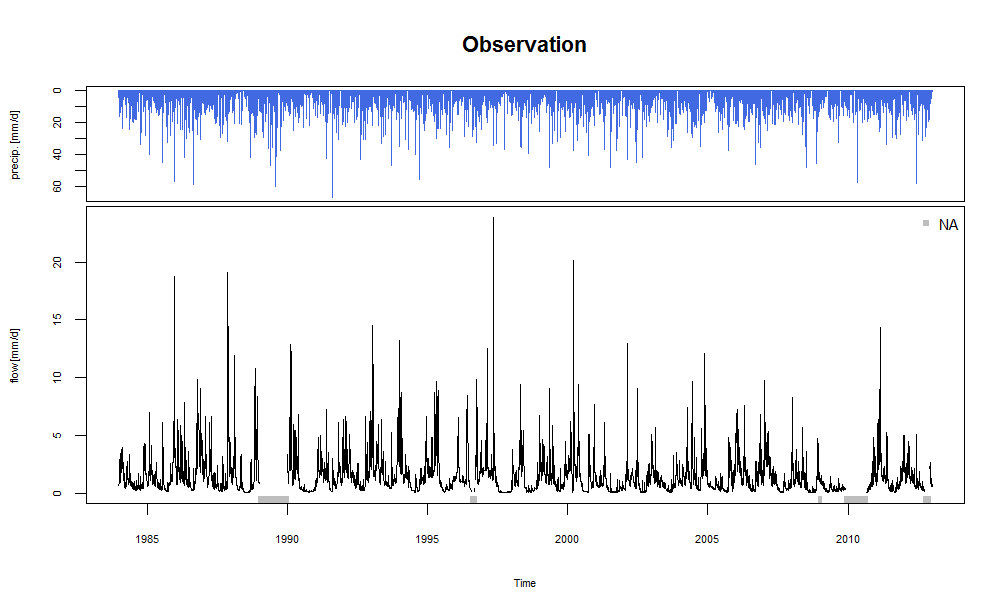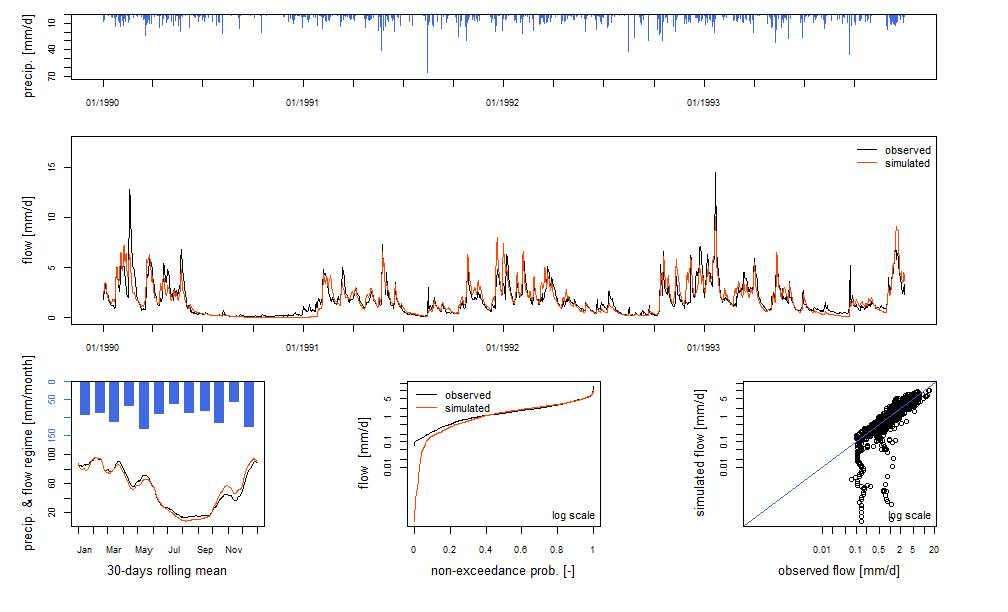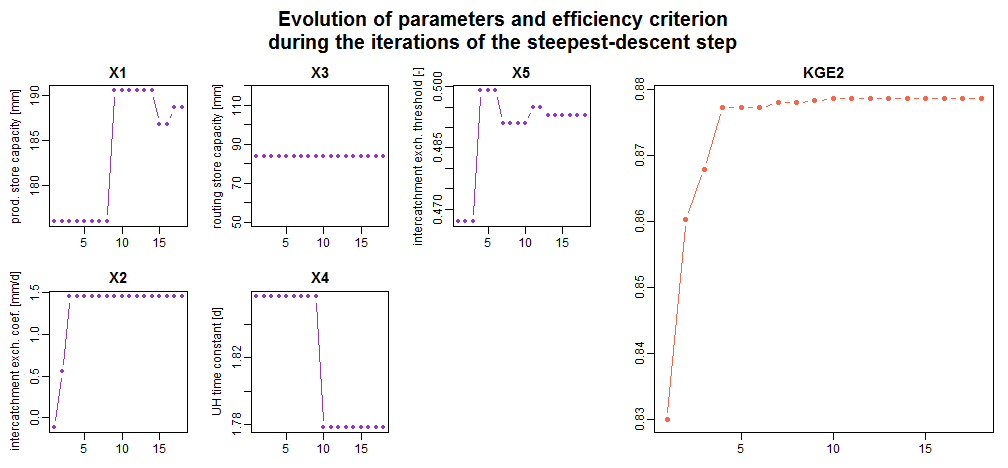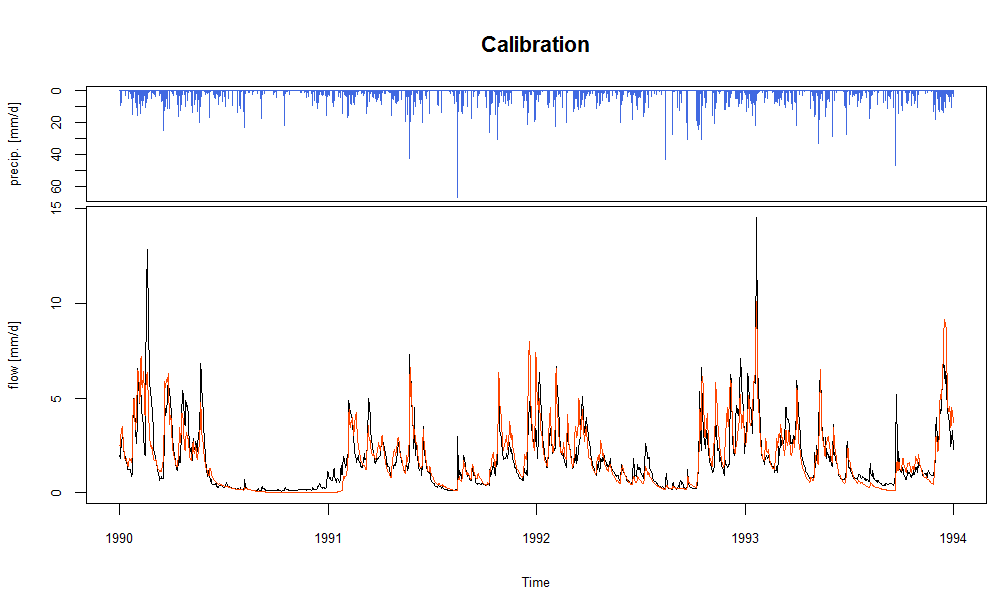# Get Started with airGRteaching

### How to run the airGR hydrological models in only three simple steps with airGRteaching

#### Preparation of input data

A data.frame of daily hydrometeorological observations time series at the catchment scale is needed. The required fields are:

• DatesR : dates in the POSIXt format
• P : average precipitation [mm/time step]
• T : catchment average air temperature [℃] [OPTIONAL]
• E : catchment average potential evapotranspiration [mm/time step]
• Qmm : outlet discharge [mm/time step]
head(BasinObs)
##       DatesR    P   E    Qmm    T
## 1 1984-01-01  4.1 0.2 0.6336  0.5
## 2 1984-01-02 15.9 0.2 0.8256  0.2
## 3 1984-01-03  0.8 0.3 2.9280  0.9
## 4 1984-01-04  0.0 0.3 1.8240  0.5
## 5 1984-01-05  0.0 0.1 1.5000 -1.6
## 6 1984-01-06  0.0 0.3 1.3560  0.9

Before running a model, airGRteaching functions require data and options with specific formats.

For this step, you just have to use the PrepGR() function. You have to define:

• ObsDF: data.frame of hydrometeorological observations time series
• HydroModel: the name of the hydrological model you want to run (GR1A, GR2M, GR4J, GR5J, GR6J or GR4H)
• CemaNeige: if you want or not to use the snowmelt and accumulation model

If you want to use CemaNeige, you also have to define:

• catchment average air temperature in ObsDF or in TempMean
• HypsoData: a vector of 101 reals: min, quantiles (1 % to 99 %) and max of catchment elevation distribution [m]; if not defined a single elevation layer is used for CemaNeige
• NLayers: the number of elevation layers requested [-]
PREP <- PrepGR(ObsDF = BasinObs, HydroModel = "GR5J", CemaNeige = FALSE)

#### Calibration step

To calibrate a model, you just have to use the CalGR() function. By default, the objective function used is the Nash–Sutcliffe criterion ("NSE"), and the warm-up period is automatically set (depends on model). You just have to define:

• PrepGR: the object returned by the PrepGR() function
• CalPer: a vector of 2 dates to define the calibration period

You can obviously define another objective function or warm-up period:

• CalCrit: name of the objective function ("NSE", "KGE", "KGE2", "RMSE")
• WupPer: a vector of 2 dates to define the warm-up period

The calibration algorithm has been developed by Claude Michel (Calibration_Michel() function in the airGR package) .

CAL <- CalGR(PrepGR = PREP, CalCrit = "KGE2",
WupPer = NULL, CalPer = c("1990-01-01", "1993-12-31"))
## Grid-Screening in progress (0% 20% 40% 60% 80% 100%)
##   Screening completed (243 runs)
##       Param =  175.915 ,   -0.110 ,   83.931 ,    1.857 ,    0.467
##       Crit KGE'[Q]      = 0.8300
## Steepest-descent local search in progress
##   Calibration completed (18 iterations, 406 runs)
##       Param =  188.670 ,    1.456 ,   83.931 ,    1.779 ,    0.493
##       Crit KGE'[Q]      = 0.8787

#### Simulation step

To run a model, please use the SimGR() function. The PrepGR and WupPer arguments of SimGR() are similar to the ones of the CalGR() function. Here, EffCrit is used to calculate the performance of the model over the simulation period SimPer and CalGR is the object returned by the CalGR() function.

SIM <- SimGR(PrepGR = PREP, CalGR = CAL, EffCrit = "KGE2",
WupPer = NULL, SimPer = c("1994-01-01", "1998-12-31"))
## Crit. KGE'[Q] = 0.8545
##  SubCrit. KGE'[Q] cor(sim, obs, "pearson") = 0.9008
##  SubCrit. KGE'[Q] cv(sim)/cv(obs)          = 0.8969
##  SubCrit. KGE'[Q] mean(sim)/mean(obs)      = 0.9737

### Pre-defined graphical plots

#### Static plots

The call of the plot() function with a PrepGR object draws the observed precipitation and discharge time series.

plot(PREP, main = "Observation")By default (with the argument which = "perf"), the call of the plot() function with a CalGR object draws the classical airGR plot diagnostics (observed and simulated time series together with diagnostic plot)

plot(CAL, which = "perf")With the CalGR object, if the argument which is set to "iter", the plot() function draws the evolution of the parameters and the values of the objective function during the second step of the calibration (steepest descent local search algorithm):

plot(CAL, which = "iter")With the CalGR object, if the argument which is set to "ts", the plot() function simply draws the time series of the observed precipitation, and the observed and simulated flows:

plot(CAL, which = "ts", main = "Calibration")The call of the plot() function with a SimGR object draws the classical airGR plot diagnostics.

plot(SIM)

#### Dynamic plots

Dynamic plots, using the dygraphs JavaScript charting library, can be displayed by the package.

The dyplot() function can be applied on PrepGR, CalGR and SimGR objects and draws the time series of the observed precipitation, and the observed and simulated (except with PrepGR objects) flows.

The user can zoom on the plot device and can read the exact values.

With this function, users can easily explore the data time series and also explore and interpret the possible problems of the calibration or simulation steps.

dyplot(SIM, main = "Simulation")

### Shiny interface

The airGRteaching package also provides the ShinyGR() function, which allows to run the Shiny interface that is proposed on this page.

The ShinyGR() function just needs:

• ObsDF: a data.frame (or a list of data.frame)
• SimPer: a vector (or list of vectors) of 2 dates to define the simulation period(s)
ShinyGR(ObsDF = BasinObs, SimPer = c("1994-01-01", "1998-12-31"))

Only daily models are currently available (GR4J, GR5J, GR6J + CemaNeige).

It is also possible to change the interface look; different themes are proposed (theme argument).